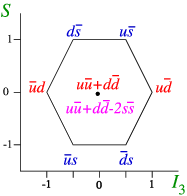$$\require{cancel}$$

# 8.3: The quark model of strong interactions

•• Contributed by Niels Walet
• Professor (Physics) at University of Manchester

Once the eightfold way (as the SU(3) symmetry was poetically referred to) was discovered, the race was on to explain it. As I have shown before the decaplet and two octets occur in the product ${\mathbf{3}} \otimes {\mathbf{3}} \otimes {\mathbf{3}} = {\mathbf{1}} \oplus {\mathbf{8} }\oplus {\mathbf{8}} \oplus{\mathbf{10}}.$ A very natural assumption is to introduce a new particle that comes in three “flavours” called up, down and strange ($$u$$, $$d$$ and $$s$$, respectively), and assume that the baryons are made from three of such particles, and the mesons from a quark and anti-quark (remember,| =.) Each of these quarks carries one third a unit of baryon number. The properties can now be tabulated (Table $$\PageIndex{1}$$).

Table $$\PageIndex{1}$$: The properties of the three quarks.
Quark label spin $$Q/e$$ $$I$$ $$I_3$$ $$S$$ $$B$$
Up $$u$$ $$\frac{1}{2}$$ $$+\frac{2}{3}$$ $$\frac{1}{2}$$ +$$\frac{1}{2}$$ 0 $$\frac{1}{3}$$
Down $$d$$ $$\frac{1}{2}$$ $$-\frac{1}{3}$$ $$\frac{1}{2}$$ -$$\frac{1}{2}$$ 0 $$\frac{1}{3}$$
Strange $$s$$ $$\frac{1}{2}$$ $$-\frac{1}{3}$$ $$0$$ $$0$$ -1 $$\frac{1}{3}$$

In the multiplet language I used before, we find that the quarks form a triangle, as given in Figure $$\PageIndex{1}$$.Figure $$\PageIndex{1}$$: The multiplet structure of quarks and antiquarks

Once we have made this assignment, we can try to derive what combination corresponds to the assignments of the meson octet, Figure $$\PageIndex{2}$$. We just make all possible combinations of a quark and antiquark, apart from the scalar one $$\eta'=u\bar{u}+d\bar{d} +c\bar{c}$$ (why?).Figure $$\PageIndex{2}$$: quark assignment of the meson octet

A similar assignment can be made for the nucleon octet, and the nucleon decaplet, see e.g., see FFigure $$\PageIndex{3}$$.Figure $$\PageIndex{3}$$: quark assignment of the nucleon octet